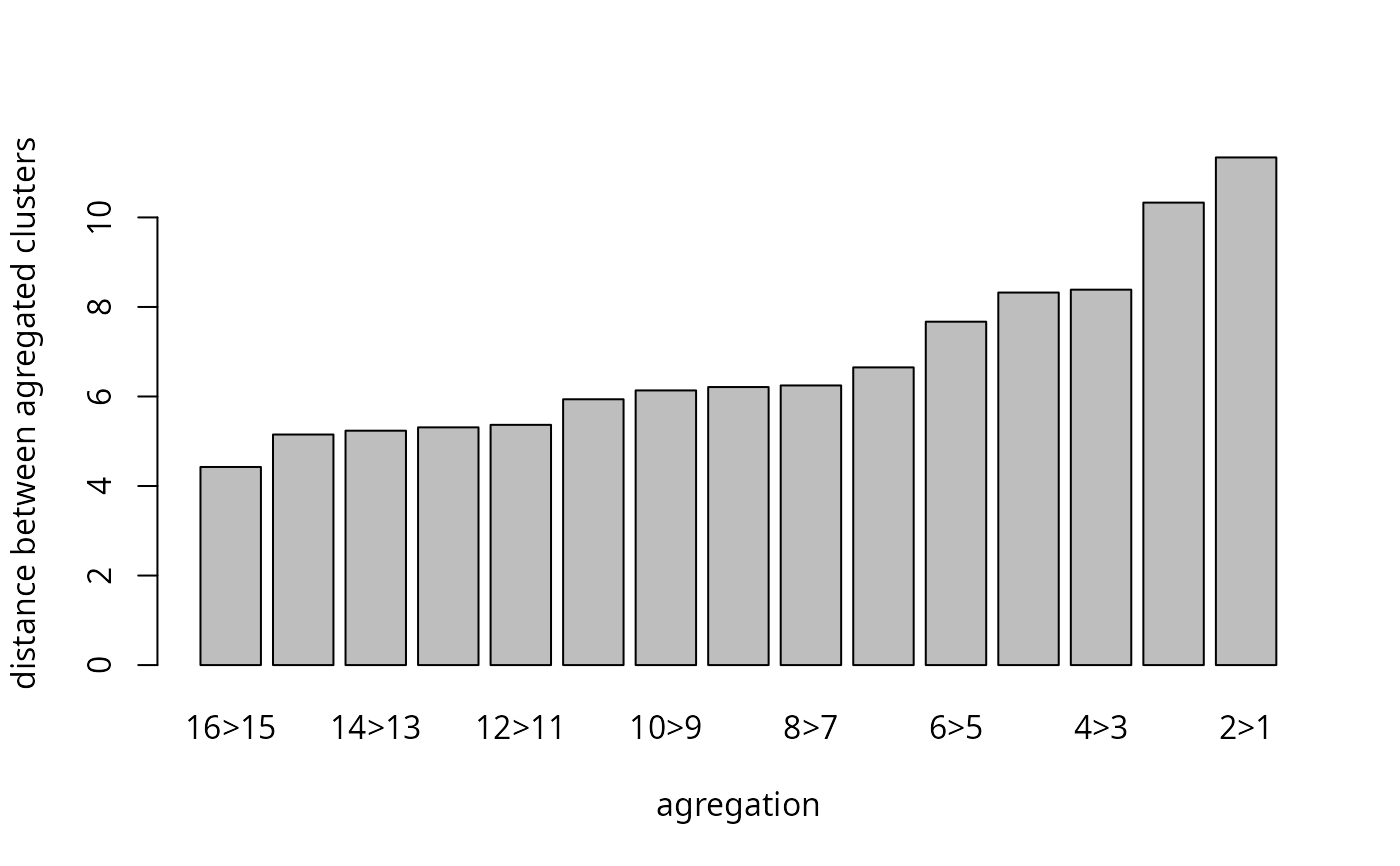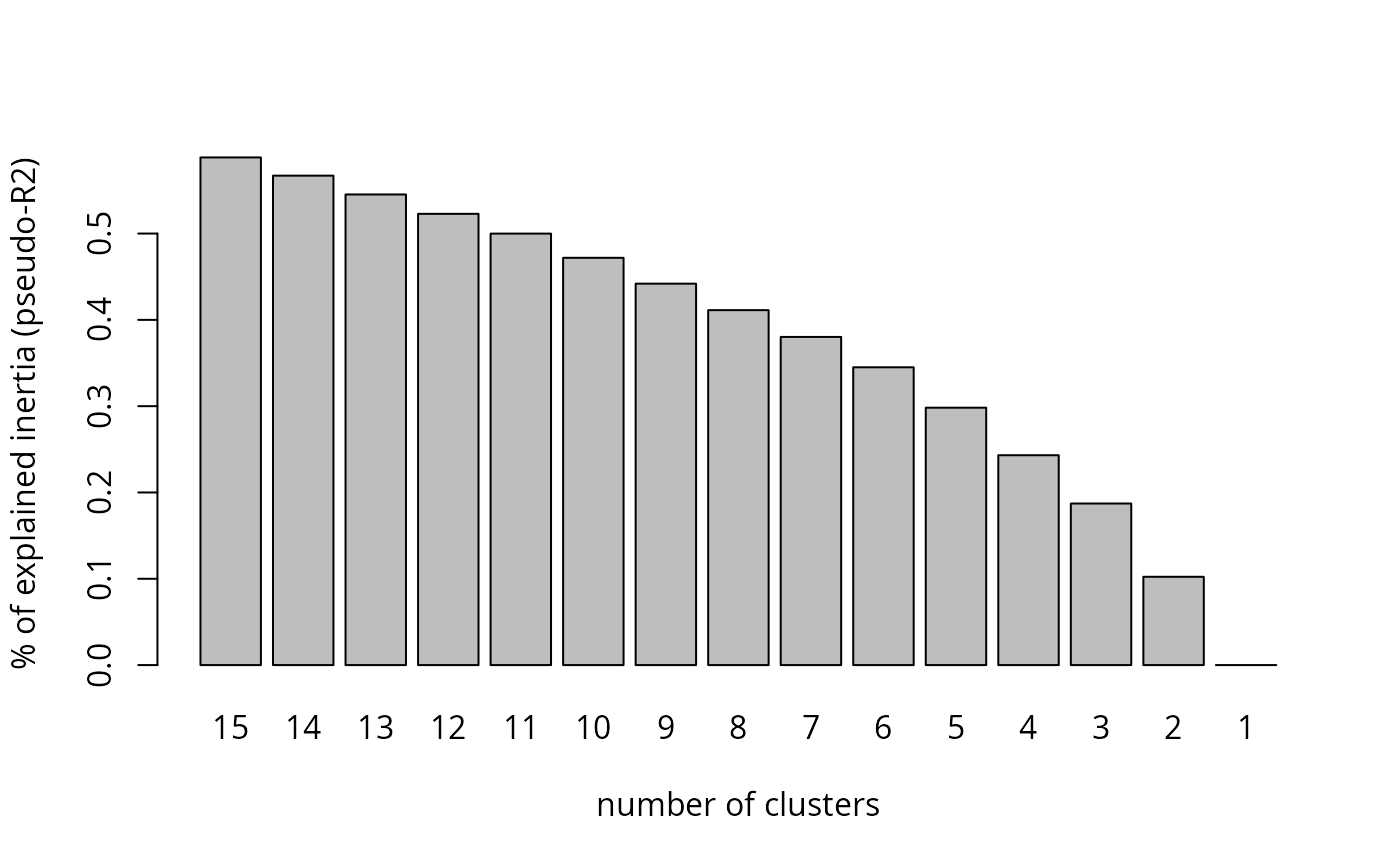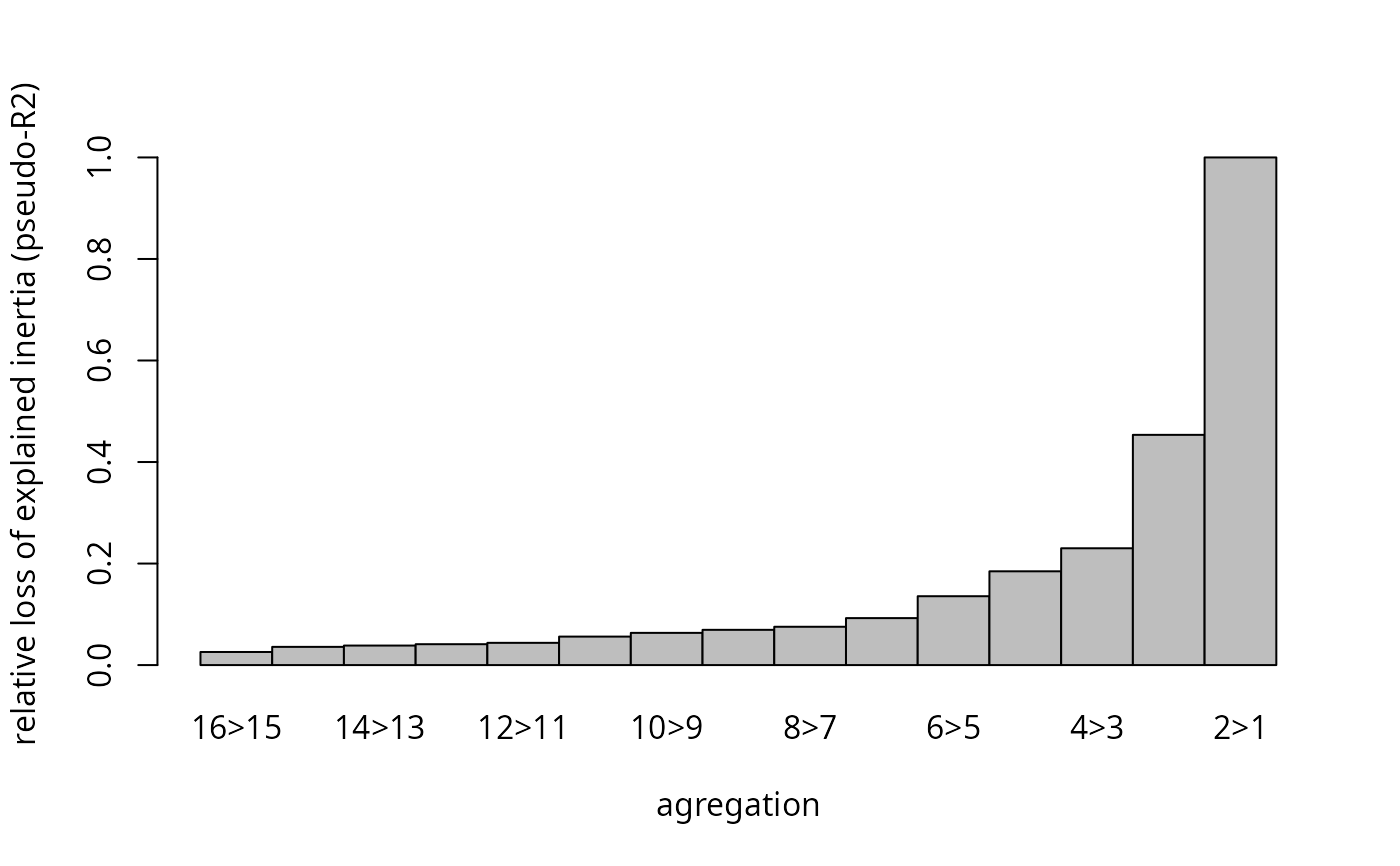Draws various plots for Ascending Hierarchical Clustering results.

ahc.plots(ahc, distance = NULL, max.cl = 20, type = "dist")

## Arguments

ahc

object of class hclust or agnes

distance

A dissimilarity matrix or a dist object. Only used if type is "inert" or "loss". Default is NULL.

max.cl

Integer. Maximum number of clusters taken into account in the plots.

type

Character string. If "dist" (default), the distance between agregated clusters is plotted. If "inert", it is the percentage of explained inertia (pseudo-R2). If "loss", it is the relative loss of explained inertia (pseudo-R2).

## Details

The three kinds of plots proposed with this function are aimed at guiding in the choice of the number of clusters.

## Author

Nicolas Robette

dist.chi2

## Examples

data(Taste)
# clustering of a subsample of the data
disjonctif <- dichotom(Taste[1:200, 1:11])
distance <- dist(disjonctif)
cah <- stats::hclust(distance, method = "ward.D2")
# distance between aggregated clusters
ahc.plots(cah, max.cl = 15, type = "dist")# percentage of explained inertia
ahc.plots(cah, distance = distance, max.cl = 15, type = "inert")# relative loss of explained inertia
ahc.plots(cah, distance = distance, max.cl = 15, type = "loss")